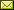## Finiteness and Computation in Toposes

 Edward Hermann Haeusler (Puc-Rio)

 Some notions in mathematics can be considered relative. Relative is a term used to denote when the variation in the position of an observer implies variation in properties or measures on the observed object. We know, from Skolem theorem, that there are first-order models where the set of real numbers is countable and some where it is not. This fact depends on the position of the observer and on the instrument/language the obserevr uses as well, i.e., it depends on whether he/she is inside the model or not and in this particular case the use of first-order logic. In this article, we assume that computation is based on finiteness rather than natural numbers and discuss Turing machines computable morphisms defined on top of the sole notion finiteness. We explore the relativity of finiteness in models provided by toposes where the Axiom of Choice (AC) does not hold, since Tarski proved that if AC holds then all finiteness notions are equivalent. Our toposes do not have natural numbers object (NNO) either, since in a topos with a NNO these finiteness notions are equivalent to Peano finiteness going back to computation on top of Natural Numbers. The main contribution of this article is to show that although from inside every topos, with the properties previously stated, the computation model is standard, from outside some of these toposes, unexpected properties on the computation arise, e.g., infinitely long programs, finite computations containing infinitely long ones, infinitely branching computations. We mainly consider Dedekind and Kuratowski notions of finiteness in this article.

In César A. Muñoz and Jorge A. Pérez: Proceedings of the Eleventh International Workshop on Developments in Computational Models (DCM 2015), Cali, Colombia, October 28, 2015, Electronic Proceedings in Theoretical Computer Science 204, pp. 61–77.
Published: 2nd March 2016.

 ArXived at: https://dx.doi.org/10.4204/EPTCS.204.6 bibtex PDF
References in reconstructed bibtex, XML and HTML format (approximated).
 Comments and questions to:eptcs@eptcs.org For website issues:webmaster@eptcs.org